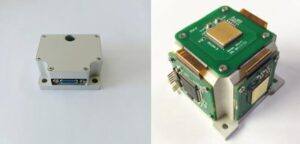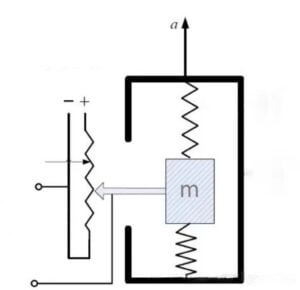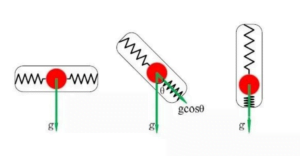Application

# Internal Structure Analysis of IMUThe full name of IMU is Inertial Measurement Unit. It is a module composed of multiple sensors such as a three-axis accelerometer and a three-axis gyroscope.

IMU is mainly used for north finding or navigation and is widely used in driverless vehicles and drones.

Let’s talk about the working principles of the three-axis accelerometer and three-axis gyroscope in Ericco’s IMU.

1. Three-axis accelerometer

Three-axis accelerometer is based on the basic principle of acceleration to achieve work.A triaxial accelerometer is an inertial sensor that can measure the specific force of an object, that is, the overall acceleration or nongravitational force acting on a unit mass without gravity. When the accelerometer remains stationary, it can sense the acceleration of gravity, while the overall acceleration is zero. In a free-fall motion, the overall acceleration is the acceleration of gravity, but the accelerometer is in a weightless state internally, and at the same time, the output of the accelerometer is zero.The three-axis accelerometer can be used to measure angles. Intuitively, as shown in the figure, the amount of spring compression is determined by the angle between the accelerometer and the ground. The specific force can be measured by the compression length of the spring. Therefore, without external force, the accelerometer can accurately measure the pitch and roll angle without accumulated error.

MEMS triaxial accelerometers use piezoresistive, piezoelectric, and capacitive operating principles, and the specific force (pressure or displacement) generated is proportional to the changes in resistance, voltage, and capacitance respectively. These changes can be collected through corresponding amplification and filtering circuits. The disadvantage of this sensor is that it is greatly affected by vibration.

2. Three-axis gyroscope

The three-axis gyroscope is the core sensitive device of the inertial navigation system, and its measurement accuracy directly affects the accuracy of the attitude calculation of the inertial navigation system.

Function: Calculate the angular velocity and the angle after integrating the angular velocity in the measurement unit.

Principle: To understand the principle of a three-axis gyroscope, one must first know the Coriolis force. Coriolis force is an inertial or fictitious force that acts on objects in motion within a frame of reference that rotates with respect to an inertial frame. The Coriolis force comes from the inertia motion of an object.

When a particle moves in a straight line relative to an inertial system, its trajectory relative to the rotating system is a curve due to its own inertia. Based on a rotating system, we believe that there is a force driving the trajectory of a particle to form a curve. Coriolis force is a description of this deviation, that is, when the motion of a straight line is placed in a rotating system, the trajectory of the straight line will shift, but the problem of actually not moving in a straight line is not affected by the force. Establishing such a virtual force is called a Coriolis force.

Therefore, in a gyroscope, we select two objects that are in constant motion and have their phases of motion differ by -180 degrees, that is, the two mass blocks move in opposite directions with the same size. The Coriolis force generated by them is opposite, thereby compressing the movement of the two corresponding capacitor plates, resulting in differential capacitance changes. The change in capacitance is proportional to the rotational angular velocity. The change in rotation angle can be obtained from the capacitance.

Ericco not only hasbut also MEMS IMU, high-precision navigation level and north-seeking level. If you are interested, please feel free to contact us.